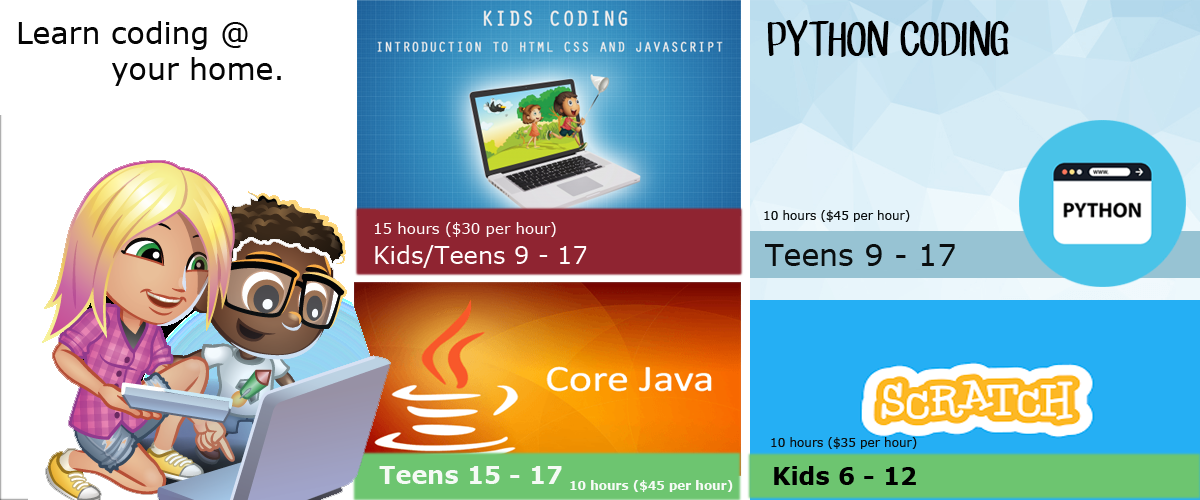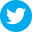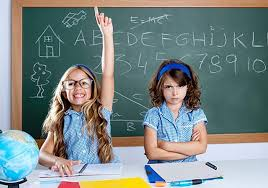Total subscribers: 4547According to a recent Channel NewsAsia commentary “The Hidden Value of Learning How To Code” by Hady W Lauw, “knowing to code may help your kid achieve an edge over others when he or she enters the workforce someday”. Let's your child learn to code and gain competitive advantage for their future. Allow your child to learn how to code at the comfort of their home. Extra \$5 will be charge for every trip made. Alternative, if you are staying near to Jurong West, you can come down to my class (Jurong West St 65). The above price tags are for 1 to 1 teaching. If you are able to get a small group of students, we can negotiate for lower price for each student. SMS/WhatApps 9877 2403 to find out more. Note: Only conduct within the vicinity of Singapore.Is Mental Math Needed Many articles and research reports have been published on the importance of mental math for children. Many educators also advocate not let children use calculator until after grade 7.

Please Share this article:Wednesday, July 06, 2016

Is Mental Math Needed

Frank Ho

BC certified math teacher

Founder of Ho Math and Chess

www.mathandchess.com

Many articles and research reports have been published on the importance of mental math for children. Many educators also advocate not let children use calculator until after grade 7. Many theories and methods teach children do fast calculation and call those calculation tips mental math. So just how much mental math do children need to acquire so they will not be at disadvantage? I have been closely watching my private students on how they do calculations and at what points they seem to be at disadvantage since the lack of their mental math ability has hampered their math ability and their marks.

My observations have been an educational experience for me since most of these so called mental math seem to have directed children into wrong ways of doing math.

I have discovered that many times children seem to run into problems is because they can not see patterns so the math IQ training is not really just to discover quick calculation tips and memorizing these rules. rather it is more helpful to them to train how to be sensitive to number patterns. For example some math weak students can not see how to do 125 to the power of 2/3 when doing this problem by hand. The calculator will not give them the answer of 5 to the power 3 is 125 so the student needs to know the power 3 of 5 is 125. Clearly the training here is to train the reverse thinking that is to train students to know that 125 has factor of 5 and then 25 has another of factor of 5.

Reversing calculations training seems to be far more important than the training of knowing some speedy math tips of multiplying 23 times 19 since student can get the product by using a calculator. For example the square root of 48 some students can not do or see 48 is a product of 3 times 16 so the answer is 4 root 3. Again here the student needs to know quickly if 48 has a perfect number factor and then use it.

It is also strange that high school students can not see 3 times 2/3 the answer is 2. The normal way for them to understand is to multiply 2 integers or 2 proper fractions multiplied together but when a integer multiplied by a fraction then they get confused. Again this has nothing to do with any mental speedy calculation but just different ways of multiplying rational numbers. The training of having students to be familiar with cross manipulation or vertical manipulation is very important.

Form above observations we can see that mental math is not limited to the scope of being a human calculator of doing some calculations in lighting speed. To acquire the mental math skills so it truly benefit students the students must learn the skill of reversing calculating like how to get factors of a number how to quickly find prime factor of a number how to find if a number has perfect factor how to not only do calculation in linear fashion but also know how to do calculations in cross or vertical way quickly and be able to see patterns. Mental math also helps student do trinomial factoring when they are in grade 9 or 10 and by the time that students find out it is difficult for them to go back to work on addition subtraction subtraction or multiplication again since it is embrasing for them to work on grade 3 math while they are already grade 10.

To increase ones math ability to train on mental math alone is not the key but to also train childrens math IQ such that the students know number symbols or figure pattern logic relation between numbers reversing calculations and different formats of calculations. By combining all these skills together they can truly increase a students thinking skills and math ability.

About the writer:  Amanda is a very experienced math teacher with B.Sc degree. Her specialty is in teaching math and chess integrated teaching as well as the integrated teaching of science and math.

Articles You might be interestedHow To Become A TutorHow To Improve Your Childs Study Skills By Discovering Their Learning StyleFive Tips To Helping Your Children With Homework